How to add hours in Excel | Productivity

# How to add hours in Excel | Productivity

Excel lets you do automated calculations to add and subtract values ​​in cells, including hours. The feature works similarly to sum of common integers, but has its peculiarities. When feeding a timesheet, for example, I need to make some adjustments so that the program does not get confused and ends up showing wrong times. Here's how to add and subtract hours without missing a count in the Microsoft spreadsheet processor.

Seven Excel Functions Little People Know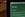Learn how to add and subtract hours to workday spreadsheet Photo: Carolina Ochsendorf / dnetc

Want to buy a cell phone, TV and other discounted products? Meet the Compare dnetc

## How to add and subtract time range

Step 1. In a time bank spreadsheet that schedules entry and exit times, mark all the cells that contain this information and access the Format cells menu;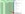Mark the cells and access the format menu Photo: Reproduction / Paulo Alves

Step 2. From the Category menu on the side, select Custom, and in the Type field type: (h): mm; @. Then press "OK" to advance and set the spreadsheet to hours;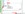Format cells to handle time data correctly Photo: Reproduction / Paulo Alves

Step 3. Normally fill in the cells with entry and exit times. In the column that marks the total hours worked on the day, use the SUM tool to subtract between the highest and the lowest hours. In the example, the formula would be: = SUM (D6 -C6);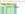Subtract the largest hour from the smallest hour Photo: Reproduo / Paulo Alves

Step 4. If the calculation has more than one hour interval, separate the shifts into different parentheses: = SUM (D6-C6) + (F6-E6). Remember to always put the time later in front of each subtraction;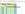Use parentheses to separate different time intervals. Photo: Reproduo / Paulo Alves

Step 5. When pressing Enter, the last column cell should display the total count of hours worked on the day;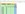D Enter to get the sum of hours worked on the day Photo: Reproduo / Paulo Alves

Step 6. Then simply click on the first cell of the count column and drag to the end to replicate the same formula to the other rows.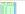Copy timekeeping formula to other lines Photo: Reproduction / Paulo Alves

## How to add and subtract amount of hours

If a time sheet only accounts for the amount of time worked, the sum can be made more directly.

Step 1. First, mark the worksheet cells that contain time data and access the format menu. Then type: (h): mm; @ in the "Type" field;Format cells to handle time data correctly Photo: Reproduction / Paulo Alves

Step 2. Create a column next to the hour count and use the sum tool to count the total amount of hours worked. In the example, just add the cells in any order.

Perform a common sum of formatted cells for hours Photo: Reproduction / Paulo Alves

How to recover text (.txt) files? Exchange tips on the dnetc forum.How to customize an Excel spreadsheet